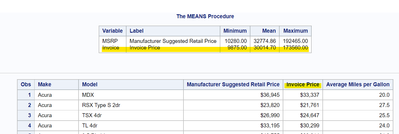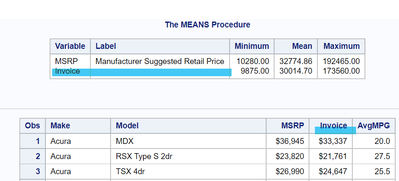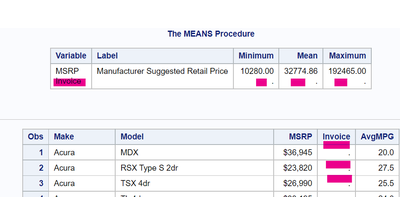## SAS Programming 1_Lesson 5_p105a03.sas: repeating the same code does not give the same outcome

Dears,

would you please clarify the reason of the following issue I encountered during the activity with p105a03.sas (SAS Studio)?

The first time everything was fine with running the code.

```data cars_update;
set sashelp.cars;
keep Make Model MSRP Invoice AvgMPG;
AvgMPG=mean(MPG_Highway, MPG_City);
label MSRP="Manufacturer Suggested Retail Price"
AvgMPG="Average Miles per Gallon"
Invoice="Invoice Price";
run;

proc means data=cars_update min mean max;
var MSRP Invoice;
run;

proc print data=cars_update label;
var Make Model MSRP Invoice AvgMPG;
run;```Due to a several days delay in my training, I wanted to refresh the knowledge and tried to complete the same task once again.

However, the outcome is not the same. Upon the original code run I can see the price values:However, upon modifying the code according to the task, the invoice value becomes empty.```
1          OPTIONS NONOTES NOSTIMER NOSOURCE NOSYNTAXCHECK;
72
73         ***********************************************************;
74         *  Activity 5.03                                          *;
75         *    1) Modify the LABEL statement in the DATA step to    *;
76         *       label the Invoice column as Invoice Price.        *;
77         *    2) Run the program. Why do the labels appear in the  *;
78         *       PROC MEANS report but not in the PROC PRINT       *;
79         *       report? Fix the program and run it again.         *;
80         ***********************************************************;
81
82         data cars_update;
83             set sashelp.cars;
84         keep Make Model MSRP Invoice AvgMPG;
85         AvgMPG=mean(MPG_Highway, MPG_City);
86         label MSRP="Manufacturer Suggested Retail Price"
87                   AvgMPG="Average Miles per Gallon";
88                   Invoice="Invoice Price";
89         run;

NOTE: Character values have been converted to numeric values at the places given by: (Line):(Column).
88:19
NOTE: Invalid numeric data, 'Invoice Price' , at line 88 column 19.
Make=Acura Model=MDX Type=SUV Origin=Asia DriveTrain=All MSRP=\$36,945 Invoice=. EngineSize=3.5 Cylinders=6 Horsepower=265
MPG_City=17 MPG_Highway=23 Weight=4451 Wheelbase=106 Length=189 AvgMPG=20 _ERROR_=1 _N_=1
NOTE: Invalid numeric data, 'Invoice Price' , at line 88 column 19.
Make=Acura Model=RSX Type S 2dr Type=Sedan Origin=Asia DriveTrain=Front MSRP=\$23,820 Invoice=. EngineSize=2 Cylinders=4
Horsepower=200 MPG_City=24 MPG_Highway=31 Weight=2778 Wheelbase=101 Length=172 AvgMPG=27.5 _ERROR_=1 _N_=2
NOTE: Invalid numeric data, 'Invoice Price' , at line 88 column 19.
Make=Acura Model=TSX 4dr Type=Sedan Origin=Asia DriveTrain=Front MSRP=\$26,990 Invoice=. EngineSize=2.4 Cylinders=4 Horsepower=200
MPG_City=22 MPG_Highway=29 Weight=3230 Wheelbase=105 Length=183 AvgMPG=25.5 _ERROR_=1 _N_=3
NOTE: Invalid numeric data, 'Invoice Price' , at line 88 column 19.
Make=Acura Model=TL 4dr Type=Sedan Origin=Asia DriveTrain=Front MSRP=\$33,195 Invoice=. EngineSize=3.2 Cylinders=6 Horsepower=270
MPG_City=20 MPG_Highway=28 Weight=3575 Wheelbase=108 Length=186 AvgMPG=24 _ERROR_=1 _N_=4
NOTE: Invalid numeric data, 'Invoice Price' , at line 88 column 19.
Make=Acura Model=3.5 RL 4dr Type=Sedan Origin=Asia DriveTrain=Front MSRP=\$43,755 Invoice=. EngineSize=3.5 Cylinders=6 Horsepower=225
MPG_City=18 MPG_Highway=24 Weight=3880 Wheelbase=115 Length=197 AvgMPG=21 _ERROR_=1 _N_=5
NOTE: Invalid numeric data, 'Invoice Price' , at line 88 column 19.
Make=Acura Model=3.5 RL w/Navigation 4dr Type=Sedan Origin=Asia DriveTrain=Front MSRP=\$46,100 Invoice=. EngineSize=3.5 Cylinders=6
Horsepower=225 MPG_City=18 MPG_Highway=24 Weight=3893 Wheelbase=115 Length=197 AvgMPG=21 _ERROR_=1 _N_=6
NOTE: Invalid numeric data, 'Invoice Price' , at line 88 column 19.
Make=Acura Model=NSX coupe 2dr manual S Type=Sports Origin=Asia DriveTrain=Rear MSRP=\$89,765 Invoice=. EngineSize=3.2 Cylinders=6
Horsepower=290 MPG_City=17 MPG_Highway=24 Weight=3153 Wheelbase=100 Length=174 AvgMPG=20.5 _ERROR_=1 _N_=7
NOTE: Invalid numeric data, 'Invoice Price' , at line 88 column 19.
Make=Audi Model=A4 1.8T 4dr Type=Sedan Origin=Europe DriveTrain=Front MSRP=\$25,940 Invoice=. EngineSize=1.8 Cylinders=4
Horsepower=170 MPG_City=22 MPG_Highway=31 Weight=3252 Wheelbase=104 Length=179 AvgMPG=26.5 _ERROR_=1 _N_=8
NOTE: Invalid numeric data, 'Invoice Price' , at line 88 column 19.
Make=Audi Model=A41.8T convertible 2dr Type=Sedan Origin=Europe DriveTrain=Front MSRP=\$35,940 Invoice=. EngineSize=1.8 Cylinders=4
Horsepower=170 MPG_City=23 MPG_Highway=30 Weight=3638 Wheelbase=105 Length=180 AvgMPG=26.5 _ERROR_=1 _N_=9
NOTE: Invalid numeric data, 'Invoice Price' , at line 88 column 19.
Make=Audi Model=A4 3.0 4dr Type=Sedan Origin=Europe DriveTrain=Front MSRP=\$31,840 Invoice=. EngineSize=3 Cylinders=6 Horsepower=220
MPG_City=20 MPG_Highway=28 Weight=3462 Wheelbase=104 Length=179 AvgMPG=24 _ERROR_=1 _N_=10
NOTE: Invalid numeric data, 'Invoice Price' , at line 88 column 19.
Make=Audi Model=A4 3.0 Quattro 4dr manual Type=Sedan Origin=Europe DriveTrain=All MSRP=\$33,430 Invoice=. EngineSize=3 Cylinders=6
Horsepower=220 MPG_City=17 MPG_Highway=26 Weight=3583 Wheelbase=104 Length=179 AvgMPG=21.5 _ERROR_=1 _N_=11
NOTE: Invalid numeric data, 'Invoice Price' , at line 88 column 19.
Make=Audi Model=A4 3.0 Quattro 4dr auto Type=Sedan Origin=Europe DriveTrain=All MSRP=\$34,480 Invoice=. EngineSize=3 Cylinders=6
Horsepower=220 MPG_City=18 MPG_Highway=25 Weight=3627 Wheelbase=104 Length=179 AvgMPG=21.5 _ERROR_=1 _N_=12
NOTE: Invalid numeric data, 'Invoice Price' , at line 88 column 19.
Make=Audi Model=A6 3.0 4dr Type=Sedan Origin=Europe DriveTrain=Front MSRP=\$36,640 Invoice=. EngineSize=3 Cylinders=6 Horsepower=220
MPG_City=20 MPG_Highway=27 Weight=3561 Wheelbase=109 Length=192 AvgMPG=23.5 _ERROR_=1 _N_=13
NOTE: Invalid numeric data, 'Invoice Price' , at line 88 column 19.
Make=Audi Model=A6 3.0 Quattro 4dr Type=Sedan Origin=Europe DriveTrain=All MSRP=\$39,640 Invoice=. EngineSize=3 Cylinders=6
Horsepower=220 MPG_City=18 MPG_Highway=25 Weight=3880 Wheelbase=109 Length=192 AvgMPG=21.5 _ERROR_=1 _N_=14
NOTE: Invalid numeric data, 'Invoice Price' , at line 88 column 19.
Make=Audi Model=A4 3.0 convertible 2dr Type=Sedan Origin=Europe DriveTrain=Front MSRP=\$42,490 Invoice=. EngineSize=3 Cylinders=6
Horsepower=220 MPG_City=20 MPG_Highway=27 Weight=3814 Wheelbase=105 Length=180 AvgMPG=23.5 _ERROR_=1 _N_=15
NOTE: Invalid numeric data, 'Invoice Price' , at line 88 column 19.
Make=Audi Model=A4 3.0 Quattro convertible 2dr Type=Sedan Origin=Europe DriveTrain=All MSRP=\$44,240 Invoice=. EngineSize=3
Cylinders=6 Horsepower=220 MPG_City=18 MPG_Highway=25 Weight=4013 Wheelbase=105 Length=180 AvgMPG=21.5 _ERROR_=1 _N_=16
NOTE: Invalid numeric data, 'Invoice Price' , at line 88 column 19.
Make=Audi Model=A6 2.7 Turbo Quattro 4dr Type=Sedan Origin=Europe DriveTrain=All MSRP=\$42,840 Invoice=. EngineSize=2.7 Cylinders=6
Horsepower=250 MPG_City=18 MPG_Highway=25 Weight=3836 Wheelbase=109 Length=192 AvgMPG=21.5 _ERROR_=1 _N_=17
NOTE: Invalid numeric data, 'Invoice Price' , at line 88 column 19.
Make=Audi Model=A6 4.2 Quattro 4dr Type=Sedan Origin=Europe DriveTrain=All MSRP=\$49,690 Invoice=. EngineSize=4.2 Cylinders=8
Horsepower=300 MPG_City=17 MPG_Highway=24 Weight=4024 Wheelbase=109 Length=193 AvgMPG=20.5 _ERROR_=1 _N_=18
NOTE: Invalid numeric data, 'Invoice Price' , at line 88 column 19.
Make=Audi Model=A8 L Quattro 4dr Type=Sedan Origin=Europe DriveTrain=All MSRP=\$69,190 Invoice=. EngineSize=4.2 Cylinders=8
Horsepower=330 MPG_City=17 MPG_Highway=24 Weight=4399 Wheelbase=121 Length=204 AvgMPG=20.5 _ERROR_=1 _N_=19
NOTE: Invalid numeric data, 'Invoice Price' , at line 88 column 19.
WARNING: Limit set by ERRORS= option reached.  Further errors of this type will not be printed.
Make=Audi Model=S4 Quattro 4dr Type=Sedan Origin=Europe DriveTrain=All MSRP=\$48,040 Invoice=. EngineSize=4.2 Cylinders=8
Horsepower=340 MPG_City=14 MPG_Highway=20 Weight=3825 Wheelbase=104 Length=179 AvgMPG=17 _ERROR_=1 _N_=20
NOTE: There were 428 observations read from the data set SASHELP.CARS.
NOTE: The data set WORK.CARS_UPDATE has 428 observations and 5 variables.
NOTE: DATA statement used (Total process time):
real time           0.00 seconds
user cpu time       0.01 seconds
system cpu time     0.00 seconds
memory              895.03k
OS Memory           30632.00k
Timestamp           03.08.2022 08:25:40 пп
Step Count                        231  Switch Count  2
Page Faults                       0
Page Reclaims                     151
Page Swaps                        0
Voluntary Context Switches        11
Involuntary Context Switches      0
Block Input Operations            0
Block Output Operations           280

90
91         proc means data=cars_update min mean max;
92             var MSRP Invoice;
93         run;

NOTE: There were 428 observations read from the data set WORK.CARS_UPDATE.
NOTE: PROCEDURE MEANS used (Total process time):
real time           0.04 seconds
user cpu time       0.03 seconds
system cpu time     0.01 seconds
memory              8555.15k
OS Memory           37308.00k
Timestamp           03.08.2022 08:25:40 пп
Step Count                        232  Switch Count  1
Page Faults                       0
Page Reclaims                     1729
Page Swaps                        0
Voluntary Context Switches        17
Involuntary Context Switches      0
Block Input Operations            0
Block Output Operations           8

94
95         proc print data=cars_update;
96             var Make Model MSRP Invoice AvgMPG;
97         run;

NOTE: There were 428 observations read from the data set WORK.CARS_UPDATE.
NOTE: PROCEDURE PRINT used (Total process time):
real time           0.66 seconds
user cpu time       0.66 seconds
system cpu time     0.00 seconds
memory              4971.00k
OS Memory           36008.00k
Timestamp           03.08.2022 08:25:41 пп
Step Count                        233  Switch Count  0
Page Faults                       0
Page Reclaims                     946
Page Swaps                        0
Voluntary Context Switches        0
Involuntary Context Switches      1
Block Input Operations            0
Block Output Operations           352

98
99
100
101
102        OPTIONS NONOTES NOSTIMER NOSOURCE NOSYNTAXCHECK;
114        ```

There is no much sense to me in the reference: "NOTE: Invalid numeric data, 'Invoice Price' , at line 88 column 19."

1 ACCEPTED SOLUTION

Accepted Solutions

## Re: SAS Programming 1_Lesson 5_p105a03.sas: repeating the same code does not give the same outcome

Hi,

You have an accidental extra semicolon in your code:

``` 82         data cars_update;
83             set sashelp.cars;
84         keep Make Model MSRP Invoice AvgMPG;
85         AvgMPG=mean(MPG_Highway, MPG_City);
86         label MSRP="Manufacturer Suggested Retail Price"
87                   AvgMPG="Average Miles per Gallon";        <--- this semicolon should not be here !
88                   Invoice="Invoice Price";
89         run;```

That ends the label statement, and then line 88 in the log becomes an assignment statement.  It is trying to assign the character value "Invoice Price" to the numeric variable Invoice.

Check out the Boston Area SAS Users Group (BASUG) video archives: https://www.basug.org/videos.
5 REPLIES 5

## Re: SAS Programming 1_Lesson 5_p105a03.sas: repeating the same code does not give the same outcome

Hi,

You have an accidental extra semicolon in your code:

``` 82         data cars_update;
83             set sashelp.cars;
84         keep Make Model MSRP Invoice AvgMPG;
85         AvgMPG=mean(MPG_Highway, MPG_City);
86         label MSRP="Manufacturer Suggested Retail Price"
87                   AvgMPG="Average Miles per Gallon";        <--- this semicolon should not be here !
88                   Invoice="Invoice Price";
89         run;```

That ends the label statement, and then line 88 in the log becomes an assignment statement.  It is trying to assign the character value "Invoice Price" to the numeric variable Invoice.

Check out the Boston Area SAS Users Group (BASUG) video archives: https://www.basug.org/videos.

Thank you.

## Re: SAS Programming 1_Lesson 5_p105a03.sas: repeating the same code does not give the same outcome

It's the pesky semi-colon on line 87:

```87                   AvgMPG="Average Miles per Gallon";
88                   Invoice="Invoice Price";
```

Because of that SAS sees Invoice="Invoice Price"; as an assignment statement.

## Re: SAS Programming 1_Lesson 5_p105a03.sas: repeating the same code does not give the same outcome

You can avoid such mistakes by using a proper visual structure where statement and terminating semicolon are on the same column:

``````label
MSRP="Manufacturer Suggested Retail Price"
AvgMPG="Average Miles per Gallon"
Invoice="Invoice Price"
;``````